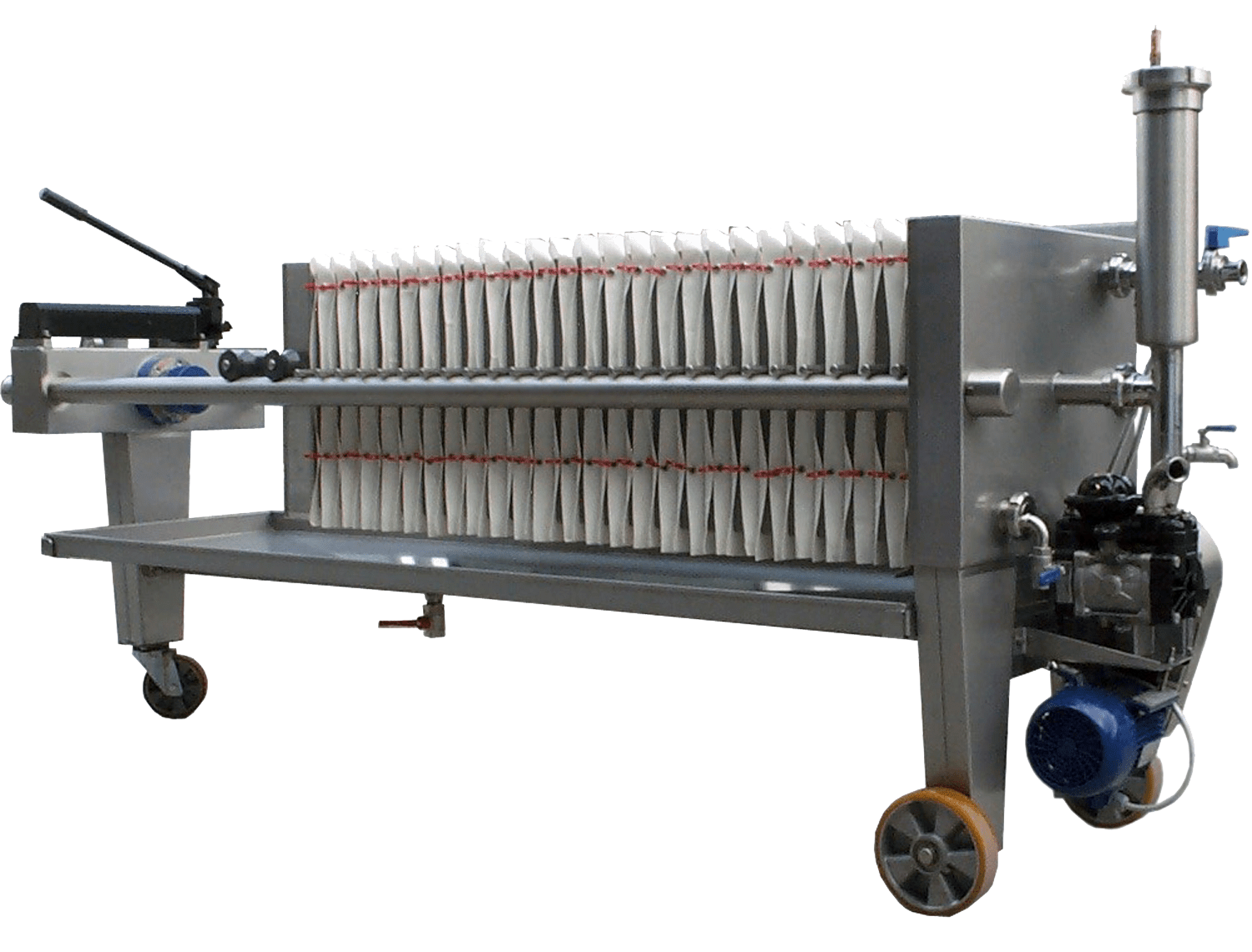## A Simple calculation on How to Determine Time Required to Obtain Certain Volume of Filtrate

In this post, I want to share how to do a simple calculation on determination of time required to obtain certain amount of filtrate in a filtration test. We will still use basic equation as in previous post.

Read previous post: How to Determine Cake Resistance in Filtration Systems

For example, we have this case.

A filter press with a surface of 50 m2

C (weight of solid per volume of liquid) = 135 kg solid/m3 liquid

μ = 0.001 N sec/m2

α (specific resistance of the cake) = 1.1 (1011) m/kg

Rf (resistance of filter cloth) = 6.5 (1010) m-1 Read More